# Reward

Three workers shared a common reward 13110 CZK: the first worker got 35% less than the second, and the third worker got 20% more than the second worker. How much got each worker?

a =  2990
b =  4600
c =  5520

### Step-by-step explanation:

a+b+c=13110
a=b-0.35b
c=b+0.20b

a+b+c=13110
a=b-0.35•b
c=b+0.20•b

a+b+c = 13110
a-0.65b = 0
1.2b-c = 0

a = 2990
b = 4600
c = 5520

Our linear equations calculator calculates it.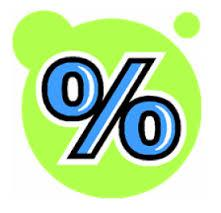Did you find an error or inaccuracy? Feel free to write us. Thank you!Tips to related online calculators
Do you have a system of equations and looking for calculator system of linear equations?

## Related math problems and questions:

• RemunerationTotal remuneration for workers is 47 000 CZK. The second worker received 20% more than the first and third 4000 CZK less than a second employee. How many CZK each received?
• Part-time workersThree part-time workers received CZK 1,235 for their work. The first received 20% less than the second and the third received 45 CZK more than the second. Determine how many crowns (CZK) each of them received.
• Czech coins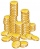John has 540 CZK, but he has 10 CZK coins and 20 CZK coins only. We know that he has 10CZK coins 7-times more than 20CZK coins. How many 10CZK coins and how many 20CZK coins did he save?
• Dividing moneyThomas, Honza and Vasek are to divide 1220kč. Honza got 25% more than Thomas. Vasek 20% less than Thomas. How CZK each got?
• Salary in enterprise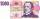The average salary in the company is 27 000 CZK, 30% of workers have the lowest average income of 19 thousand CZK. There were an increase in the salary in this group by 2%. How much % increased the average salary across the company?
• ContestantsThe three best contestants are to divide the total prize of CZK 4,200. The second gets 20% more than the third. And the first one gets 200 CZK less than the second and the third together. How much will everyone get?
• Average monthly salaryA total of 10 teachers work at one small school in Moravia. The monthly salary of each is 21,500 CZK or 21,800 CZK or 22,500 CZK according to their education and age. The average monthly salary for this school's teacher is 21 850 CZK. How many teachers of
• Three friendsThree friends divided the profit by 104,650 CZK, so that for every 4 CZK, which got the first friend equals 5 crowns for second and for every 9 CZK, which got the second equals 16 CZK for third. Question: Who got the most and how much.
• Three workers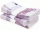The three workers received € 2,850 together for the work done. They divided them according to the time worked so that the first received 20% less than the second and the third € 50 more than the second. How much EUR did each worker receive?
• Purchase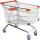Mother bought 5 boxes of milk and 7 kg of potatoes and paid a total CZK 147. Aunt bought 7 boxes of milk and 3 kg of potatoes and paid 131 CZK. What is the price of one carton of milk and 1 kg of potatoes? How CZK together would have saved if bought at th
• Performance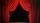Same number of tickets sold for 15 CZK, 20 CZK, 30 CZK and 40 CZK a total of CZK 13,230 on theatre performance. How many tickets were sold for this performance?
• Eva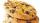When Eva buys 8 packages of cookies, 4 CZK left her. If she wanted to buy 10 packages, she would have to borrow 20 CZK. How much money has Eva in her wallet?
• Cost reductionTwo MP3 players whose price was equal to originally have been discounted the first by 20%, the second by 35%. The difference in their prices 750 CZK was after the price reduction. What was the original price of each of the two players?
• Scouts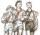Scouts purchased for camp cans two types total cost 1460 CZK. The first cans were for CZK 32 and the second for 25 CZK. How many cans purchased for each type?
• Three workersThe company rewarded three workers CZK 9200, and the money was divided by the work they have done. The first worker to get twice than the second, the second three times more than the third. How much money did each worker receive?
• Siblings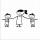Three siblings had saved up a total of 1,274 CZK. Peter had saved up to 15% more than Jirka and Hanka 10% less than Peter. How much money did they save each one of them?
• Birthday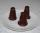Mother bought 21 desserts on the occasion of Mirka's birthday one tips was 9 CZK and the kremlin cost 12 CZK. For all desserts, she paid 213 CZK. How many kremlins and how many tips mums did buy?